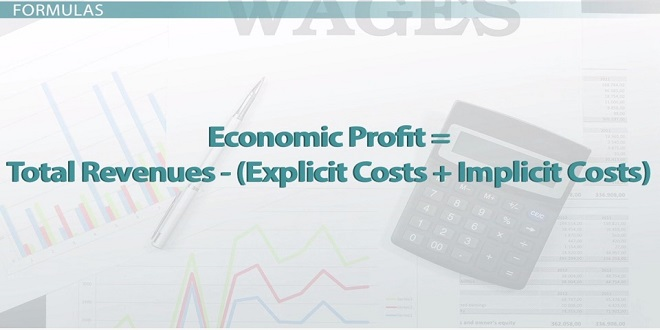# Profit – Definition & Formula

Profit is a phrase that almost all of us use on a regular basis. Several times in your life, you may have been in a monetary profit or loss position. We employ the profit formula on a regular basis without much consideration. In this post, we will attempt to discover the profit formula, as well as some key terminology associated with it, like cost price and selling price, as well as the derivation of the profit formula as well as some instances. We’ll also look at how Cuemath may help you succeed in these subjects and strengthen your mathematical foundation.

In this article, we are going to discuss profit definition and profit formula.

## Profit Formula

For any business or work to continue a person needs to have profits. A man has a profit when the selling price of any object is more than the cost price of the object. If the cost price of the object is more than the selling price of the object then the man has a loss.

Selling price is defined as the price at which the item is being sold. Cost price is defined as the price at which an item is being bought. The selling price is denoted as S.P and the cost price is denoted as C.P.

Profit Formula = Selling Price (S.P) – Cost Price (C.P)

Let’s go through a small example, Rahul has a shop where he sells one dozen eggs for rupees 120 whereas the cost price of buying one dozen eggs is rupees 150.

We can see that the selling price is less than the cost price for a dozen eggs. Therefore, it is clear that Rahul had a loss. Since Cost Price > Selling Price, Loss = Cost Price – Selling Price which when calculated will be equal to a loss of 30 rupees.

## Solved Examples Related to Profit Formula

Example 1: Nirmal is trying to sell an item she bought from a local store at rupees 50. She has decided to sell the item at rupees 120. Did Nirmal have a loss or a profit?

Solution: Let’s list down all the information given in the question,

The selling price of the item = 120 rupees

The cost price of the item = 50 rupees

We can see that the SP> CP, which means Nirmal has a profit. Let’s calculate the profit. We have already discussed the profit formula,

Profit will be equal to S.P – C.P (S.P is selling price and C.P is the cost price)

120 rupees – 50 rupees = 70 rupees

Thus, by selling the item at rupees 120, Nirmal made a profit of 70 rupees.

Example 2: Ram buys chocolate for rupees 10 and sells the chocolate at rupees 12. What is the profit earned by Ram in the transaction?

Solution: S.P = Rs.12

C.P = Rs 10

Profit = S.P – C.P =12-10 = Rs. 2 is the profit.

## Learn from Math Online Classes

Mathematics is known to be a subject that is considered to be logical and there are various aspects that require assistance and guidance from the teachers. Do you have a problem studying math? Yes. I feel that the problem is not with you nor with the subject. The problem must be with your teacher. Hence, you must visit Cuemath. It provides you with teachers who are highly qualified and experienced. Not only the teachers also help you by providing math puzzles, math games, and math worksheets which increases the rate of rationalized reasoning and logic within yourself. Hence, Visit Cuemath’s website and master the subject from math online classes. Go and book a free session now.